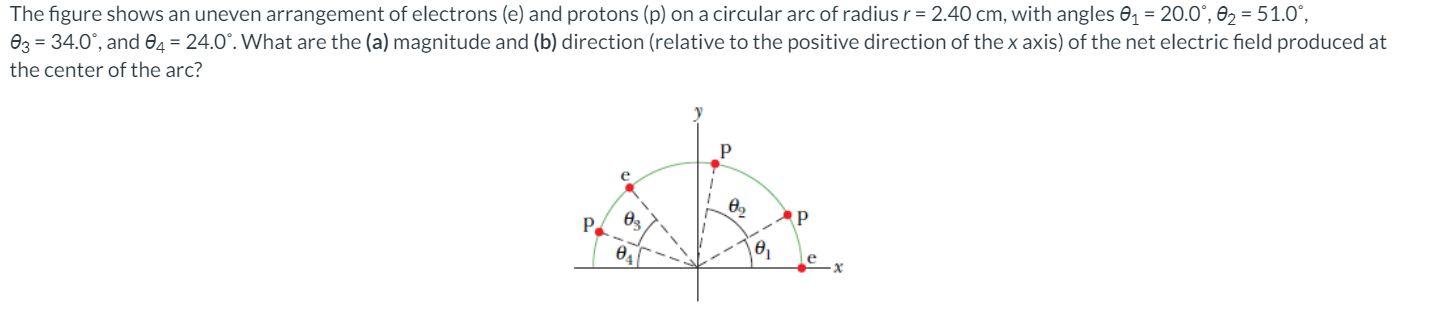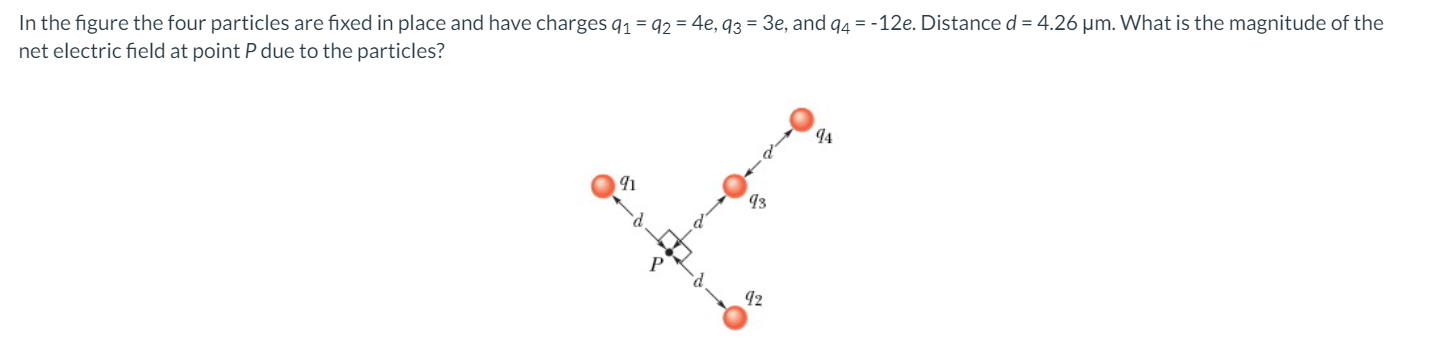# (Solved): The Figure Shows An Uneven Arrangement Of Electrons (e) And Protons (p) On A Circular Arc Of Radius ...

Question 83)

PART 1)PART 2)The figure shows an uneven arrangement of electrons (e) and protons (p) on a circular arc of radius r = 2.40 cm, with angles 01 = 20.0Â°, 02 = 51.0Â°, 03 = 34.0Â°, and 04 = 24.0Â°. What are the (a) magnitude and (b) direction (relative to the positive direction of the x axis) of the net electric field produced at the center of the arc? In the figure the four particles are fixed in place and have charges 91 = 92 = 4e, 93 = 3e, and 94 = -12e. Distance d = 4.26 um. What is the magnitude of the net electric field at point P due to the particles? 42

We have an Answer from Expert